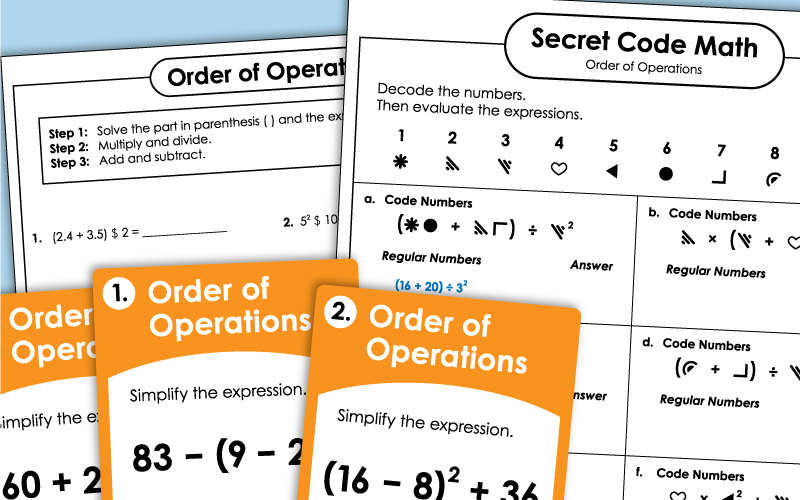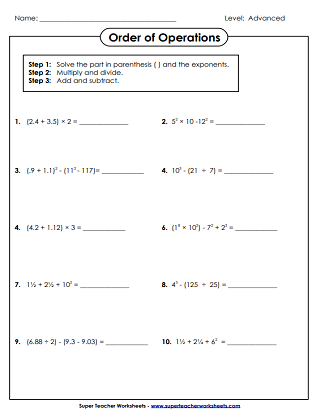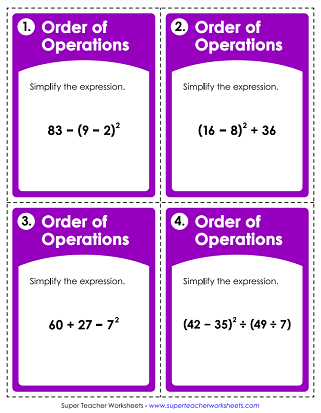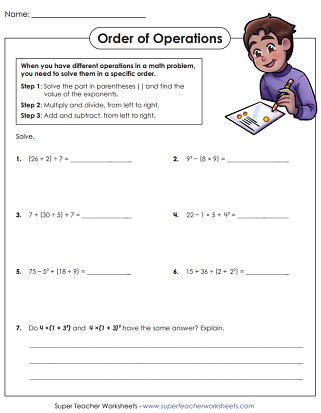# Order of Operations Worksheets (Includes Parenthesis & Exponents)

On these worksheets, students will evaluate expressions and solve equations using the order of operations. This page contains advanced level activities that include parenthesis and exponents.## Whole Numbers Only

Solve the equations using order of operations. Includes parentheses, exponents. Whole numbers only.
This file contains 30 task cards with expressions. Expressions contain parenthesis. Students use their knowledge of Order of Operations to simplify each.
example: 83 - (9 - 2)²
These problems include basic parenthesis and exponents. There is a short answer question included.
Use the cryptogram symbol key at the top of the page to decode the expressions. Then simplify (solve) each.
Color each problem, as well as the answer. If you complete them all, you'll be able to navigate the maze from start to finish.

## Includes Fractions & Decimals

Solve the equations. These problems include parentheses and exponents. Decimals and fractions also included.
More Order of Operations

Follow this link to see all different levels of activities for teaching Order of Operations.

## Sample Worksheet ImagesMy Account
Site Information